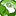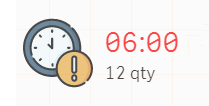# New to Qlik Sense

If you’re new to Qlik Sense, start with this Discussion Board and get up-to-speed quickly.

Announcements
cancel
Showing results for
Did you mean:Contributor II

## sum distinct with unique data

I've been looking for similar issue, but i'm getting confused with the keyword

so, I have this table

 type time quantity type_A 06:00 10 type_B 07:00 4 type_C 06:00 2 type_A 07:00 5

I want to sum the quantity based on time and ignore the type. (I'm using type there so if i use "type" as filter, the quantity will be changed).

my issues are if I load the table like that, the sum result is not correct. But if I didn't load the "type" column, the quantity (in KPI) won't change based on the filter which is "type" but the sum results is correct.

the result that i want is a table like this:

 time quantity 06:00 12 07:00 9

I want to use the new table to display the time with highest quantity (as a KPI chart) and add comment with the quantity

the current expression I'm using is: FirstSortedValue(distinct time, aggr(sum(quantity),time))

Labels (4)

• ### newbie

13 RepliesSpecialist

Try this
aggr(sum(quantity),time)Contributor II
Author

I tried it, it works fine in a table, but it didn't work in KPI, the result is "-".

i'm afraid that the type is blocking this operation because when i select the distinct data for time, the type couldn't be combined (because it's different every row).SpecialistContributor II
Author

Actually, I want to display a KPI Chart about Time that has most Quantity & display the quantity as comment on that KPI chart.

so from the example data i wrote above, the expected result  is like this:(I updated my original question with more explanation of my expected result).Specialist

Try this for Quantity

MAX(aggr(sum(quantity),time))

Try this for time
=FirstSortedValue(time, -aggr(sum(quantity),time))MVP

For max quantity use below

MAX(aggr(sum(quantity),time))

For max Time use below

Firstsortedvalue( distinct time, -aggr(sum(quantity),time))Contributor II
Author

Hi, thank you! adding the "Max" is really works.
But actually i still have problem with this..

if i use [type] as filter, the quantity didn't change based on the type.

for example:

when I choose type = type_c, the quantity should be "2" instead of "12" (the total qty of time 06:00)

is there any solution for this? i tried this: MAX(aggr(sum(quantity),time,type)) but it still didn't workCreator III

Hello,

MAX(aggr(sum(quantity),time,type))  won't work because you want to aggregate your data towards a time and not the type, right ? Othterwise you also won't get 12 for the first output.

As you can see a KPI Window cannot express more than one output. So you got the wanted output after you add a Max() function, because it rightly tells you only to display the MAX VALUE which is unique.Creator III

Maybe try to work with a "filterwindows" where you can choose the  right type you want to display.

It should display the total value of the type you choose in the filerwindow.

Like this : sum(quantity) and the time in another kpi window.

thats imho the easiest way to solve that dynamically.

Did I miss something?